### SUPPORT VECTOR MACHINES:

From this MIT video.

It is predicated upon drawing a decision boundary line leaving as ample a margin to the first positive and negative examples as possible: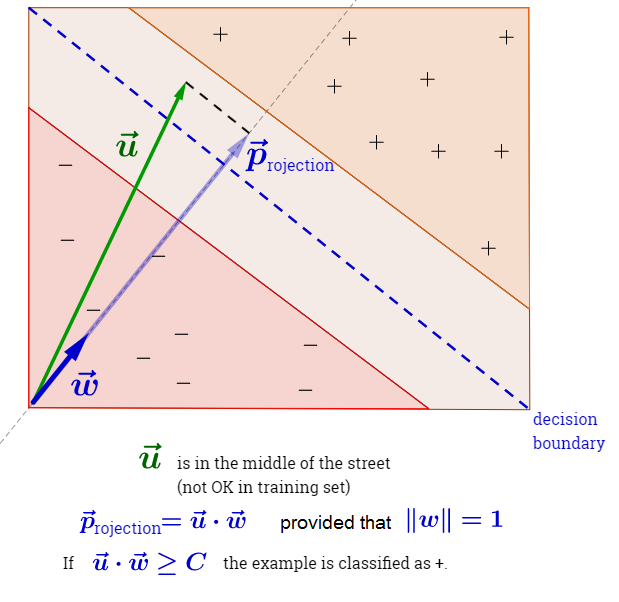As in the illustration above, if we select an orthogonal vector such that $$\large \lVert w \rVert=1$$ we can establish a decision criterium for any unknown example $$\bf u$$ to be catalogued as positive of the form:

$$\large \color{blue}{w} \cdot {\bf u} \geq c$$, corresponding to a value that would place the projection beyond the decision line in the middle of the street. Notice that $$\color{blue}{w} \cdot {\bf u} = {\bf u} \cdot \color{blue}{w}$$.

An equivalent condition would be:

$\Large\color{blue}{w}\cdot u + b \geq 0 \tag{decision line or rule: Eq.1}$

with $$c = - b$$ the sample is positive.

So we need $$b$$ and $$\color{blue}{w}$$ to have a decision rule. To get there we need constraints.

First constraint we are going to impose is that for any positive sample $$x_+$$ (we could have used $$u_+$$), $$\color{blue}{w}\cdot \bf x_+ + b \geq 1$$; and for negative samples, $$\color{blue}{w}\cdot \bf x_- + b \leq -1$$.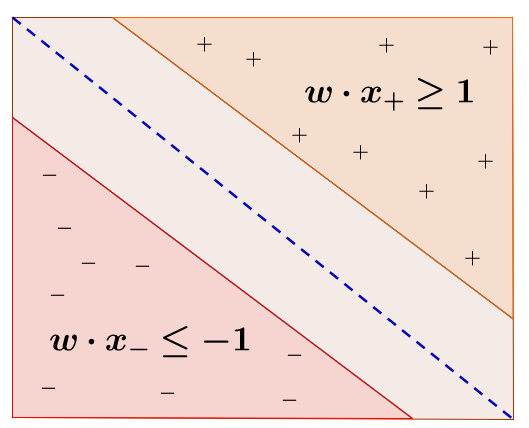In the division boundary or hyperplane (median) the value would be $$0$$, leaving the values of the different strips as: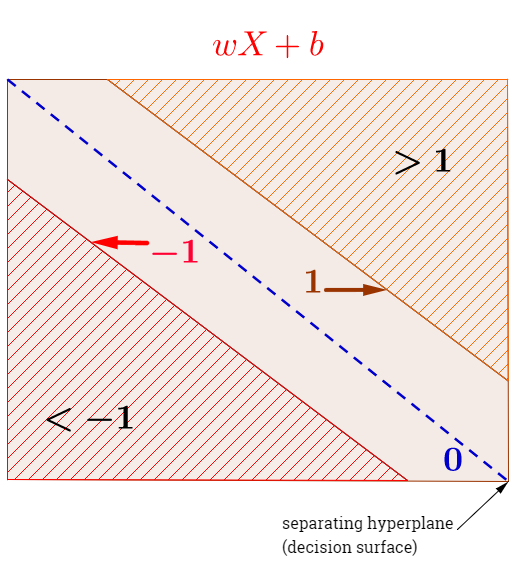The vector $$\bf w$$ is the weight vector, whereas $$b$$ is the bias.

To bring these two inequalities together, we can introduce the variable $$y_i$$ so that $$y_i=+1$$ for positive examples, and $$y_i=-1$$ if the examples are negative, and conclude $$\large y_i (x_i\cdot \color{blue}{w} + b) -1\geq 0$$.

So we establish that this has to be greater than zero, but if the example is on the hyperplanes that maximize the margin of separation between the decision hyperplane and the tips of the support vectors, in this case a lines), then:

$\Large y_i \,(x_i\cdot \color{blue}{w} + b) -1 = 0\tag{Eq.2}$

Notice that this is equivalent to requiring that $$y_i \,(x_i\cdot \color{blue}{w} + b) = 1.$$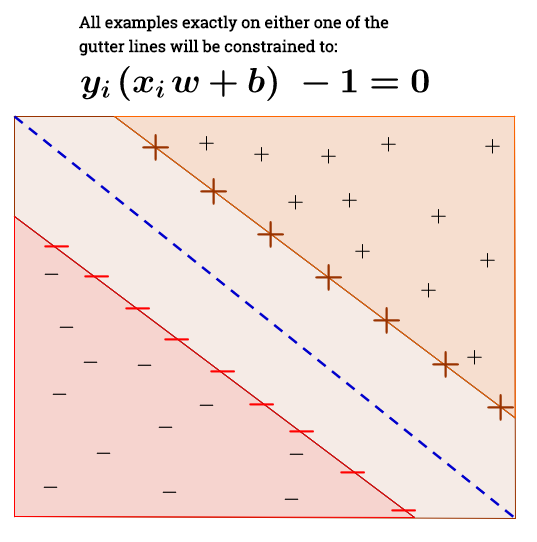Second constraint: the distance of the decision hypeplane to the tips of the support vectors will be maximized. In other words the margin of separation (“street”) will be maximized: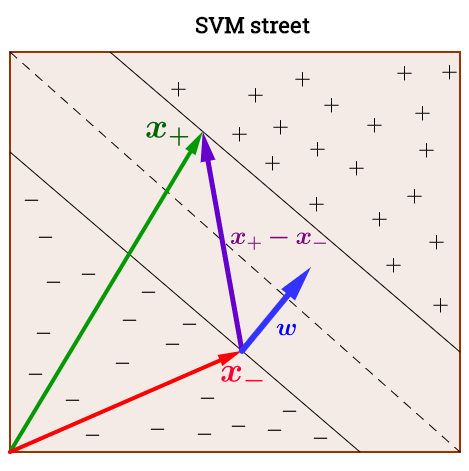Again, assuming a unit vector perpendicular to the decision boundary, the dot product with the difference between two “bordering” plus and minus examples is the width of “the street”:

$\large \text{width}= (x_+ \,{\bf -}\, x_-) \cdot \frac{w}{\lVert w \rVert} \tag{the width of the street}$

Now, key observation: on the equation above $$x_+$$ and $$x_-$$ are in the gutter (on hyperplanes maximizing the separation)! Therefore, for the positive example:

$$\large ({\bf x_i}\cdot \color{blue}{w} + b) -1 = 0$$, or $$\large {\bf x_+}\cdot \color{blue}{w} = 1 - b$$; and for the negative example: $$\large {\bf x_-}\cdot \color{blue}{w} = -1 - b$$. So, reformulating the width of the street:

\begin{align}\text{width}&=(x_+ \,{\bf -}\, x_-) \cdot \frac{w}{\lVert w \rVert}\\&= \frac{x_+\cdot w \,{\bf -}\, x_-\cdot w}{\lVert w \rVert}\\&=\frac{1-b-(-1-b)}{\lVert w \rVert}\\&= \frac{2}{\lVert w \rVert} \tag{the width of the street: Eq.3}\end{align}

So now we just have to maximize the width of the street - maximize $$\large \frac{w}{\lVert w \rVert}$$ (or maximize $$\Large \frac{2}{\lVert w \rVert}$$, or minimize $$\Large \lVert w \rVert)$$, or minimize:

$\large \text{min}\frac{1}{2}\lVert w \rVert^2 \tag{minimization objective: Eq.4}$

which is mathematically convenient.

So we want to:

1. Minimize $$\lvert x\rvert^2$$ with the constraint:

2. $$y_i(x_i\cdot w + b)-1=0$$

So we want to minimize this expression based on some constraints. Hence we need a Lagrange multiplier (going back to equations 2 and 4):

$\large \mathscr{L} = \frac{1}{2} \lVert w \rVert^2 - \sum \lambda_i \Big[y_i \, \left( x_i\cdot \color{blue}{w} + b \right) -1\Big]\tag{Lagrange: Eq.5}$

Differentiating,

$$\large \frac{\partial \mathscr{L}}{\partial \color{blue}{w} }= \color{blue}{w} - \sum \lambda_i y_i x_i = 0$$. Therefore,

$\large\color{blue}{w} = \sum \lambda_i \,\, y_i \,\, x_i\tag{Orthonormal vector: Eq.6}$

$$\large \frac{\partial \mathscr{L}}{\partial b}=-\sum \lambda_i y_i = 0$$, which means that

$$\large \sum \lambda_i \, y_i = 0\tag{zero sum multipliers times labels: Eq.7}$$

Pluging equation [Eq.6] back into [Eq.5],

$\mathscr{L} = \frac{1}{2} \color{purple}{\left(\sum \lambda_i y_i x_i \right) \,\left(\sum \lambda_j y_j x_j \right)}- \color{green}{\left(\sum \lambda_i y_i x_i\right)\cdot \left(\sum \lambda_j y_j x_j \right)} - \sum \lambda_i y_i b +\sum \lambda_i$

The penultimate term is zero as per equation [Eq.7].

Therefore,

$\bbox[15px,border:2px solid blue]{\large \mathscr{L} = \sum \lambda_i - \frac{1}{2}\displaystyle \sum_i \sum_j \lambda_i \lambda_j\,\, y_i y_j \,\, x_i \cdot x_j}\tag{final Lagrangian: Eq.8}$

Hence, the optimization depends on the dot product of pairs of examples.

Going back to the “decision rule” equation [Eq.1] above, and using [Eq.6]:

$\large \sum \lambda_i y_i x_i\cdot u + b \geq 0\tag{final decision rule: Eq.9}$